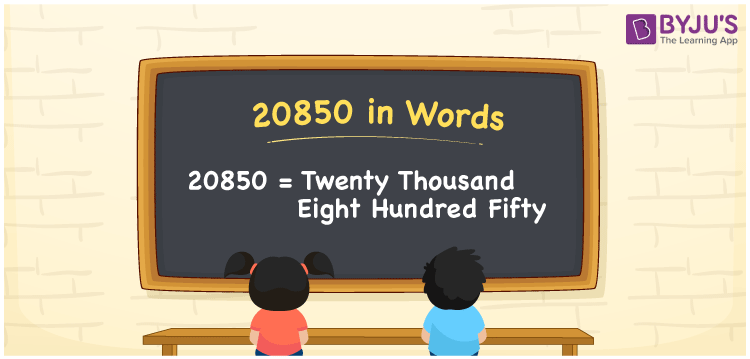# 20850 in words

20850 in words is written as Twenty Thousand Eight Hundred and Fifty. In 20850, 2 has a place value of ten thousand, 8 is in the place value of hundred and 5 is in the place value of ten. The article on Place Value gives more information. The number 20850 is used in expressions that relate to money, distance, social media views, and many more. For example, “There are Twenty Thousand Eight Hundred and Fifty new voters that need entry in the list.”

 20850 in words Twenty Thousand Eight Hundred and Fifty Twenty Thousand Eight Hundred and Fifty in Numbers 20850

## 20850 in English Words## How to Write 20850 in Words?

We can convert 20850 to words using a place value chart. The number 20850 has 5 digits, so let’s make a chart that shows the place value up to 5 digits.

 Ten thousand Thousands Hundreds Tens Ones 2 0 8 5 0

Thus, we can write the expanded form as:

2 × Ten thousand + 0 × Thousand + 8 × Hundred + 5 × Ten + 0 × One

= 2 × 10000 + 0 × 1000 + 8 × 100 + 5 × 10 + 0 × 1

= 20850.

= Twenty Thousand Eight Hundred and Fifty.

20850 is the natural number that is succeeded by 20849 and preceded by 20851.

20850 in words – Twenty Thousand Eight Hundred and Fifty.

Is 20850 an odd number? – No.

Is 20850 an even number? – Yes.

Is 20850 a perfect square number? – No.

Is 20850 a perfect cube number? – No.

Is 20850 a prime number? – No.

Is 20850 a composite number? – Yes.

## Solved Example

1. Write the number 20850 in expanded form

Solution: 2 x 10000 + 0 x 1000 + 8 x 100 + 5 x 10 + 0 x 1

We can write 20850 = 20000 + 0000 + 800 + 50 + 0

= 2 x 10000 + 0 x 1000 + 8 x 100 + 5 x 10 + 0 x 1.

## Frequently Asked Questions on 20850 in words

Q1

### How to write the number 20850 in words?

20850 in words is written as Twenty Thousand Eight Hundred and Fifty.
Q2

### Is 20850 a prime number?

No. 20850 is not a prime number.
Q3

### Is 20850 divisible by 10?

Yes. 20850 is divisible by 10.